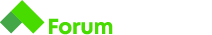# Нужда от помощ за Data Types and Variables Coding Tasks : Interest

Здравейте приятели. Бихте ли ми казали къде бъркам . Ето го и условието и моето решение и ви благодаря предварително# Interest

You have deposited a sum into your bank account for 3 years. The bank has announced an interest of 5% per year. Each time the interest is calculated and added to your deposit. You have to calculate the amount in your deposit for each year.

## Input

• On the only line you will receive a number (n) with the sum deposited

## Output

• You should print the amount in your deposit for each of the 3 years

## Constraints

• You must print the number with two numbers after the decimal point. The rules of math for rounding apply here

## Input

``````1000
``````

let print = this.print || console.log;

// Use gets() to receive one line of input (the input line is always a string)
// Use “print” instead of “console.log” to print the result
let input = 1000;
let depositedSum = +gets();
let depositeOne = (5/100) * depositedSum;
let depositeTwo = (5/100) * depositeOne;
let depositeThree = (5/100) * depositeTwo;
let totalSum = (depositeOne + depositeTwo + depositeThree);
print(totalSum.toFixed(2));

Здравей,
Изчислението и печатането е грешно.
Прочети отново условието по-подробно. Ако е необходимо, прегледай отново инструкциите как се решават задачи тук и тук.
Oчаква се да отпечаташ сумата за всяка една от трите години.
За Input (входни данни) : 1000
Се очаква Output (да се отпечата)

``````1050.00
1102.50
1157.63
``````

В таба “my submissions” можеш да видиш какво е отпечатало твоето решение.

1 Like

Благодаря , че ме упътихте. Нов съм в това и още не ги чаткам. Мисля ,че разбрах кой метод трябва да използвам. Не знам защо така съм се подвел. Живи и здрави

1 Like